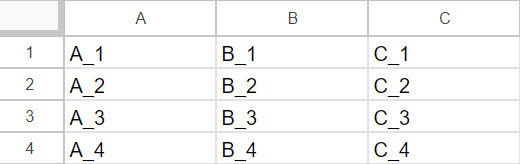# Objective as a function of constrains

Hello,

I am trying to build a CQM where the objective depends on conditions. I have a table of time-dependent entries labeled A, B, and C. (Such that entry1 = (t0, 1(A), 2(B), 2(C)), entry2 = (t1, -3,4,-1), with a large n number of entries) ) I wish to formulate the objective to minimize R, which is found by iterating through the table and checking if A > alpha and B > beta, and if both conditions are met, add C to the rolling sum R. Specifically, I want to tune alpha(an Integer object with a predefined range) and beta to minimize R. Additionally, I hope to generalize the form to expand beyond 2 conditions(where all conditions must be satisfied to add C to R).

for entry in entries:

if (entry.A > x and entry.B > x): #where x = alpha and x = beta

R+=entry.C

Wiktor

• Hi Wiktor,

Is it possible to elaborate more on how entry is defined? A printout of an entry would also be nice.

To formulate this, you can do binary arithmetic on Booleans for each condition but ensure everything is still quadratic.

We can start by defining binary variables Xi and Yj to indicate whether the conditions(entryi.A>α) and entryj.B>β) are satisfied for ith and jth entries respectively, and any other entries added to it.

Something along the line of:Please let me know if you can provide a bit more details on how `entry` is defined.

Best Regards,
Tanjid

• Hi Tanjid,

Thanks for the response!

An entry is represented as a tuple (A_t, B_t, C_t). For example, at t=0: (1,2,-3). These are all defined in the following table.Your suggestion is a good way to compute R, but this approach involves recomputing the variables X and Y at each iteration. I am not too familiar with CQM, so would this objective definition be a problem since the table would need to be accessed and the variables X and Y would still need to be computed with a conditional statement? Also, I only care about the "same entry conditions", so R would be defined with only a single summation from i=1 to n with the 2nd summation removed(replace j with i).

Best,
Wiktor

• Hi Wiktor,

Do you have a code snippet that you would be able to share, where X and Y are being defined? Can you elaborate more on what do you mean by recomputing X and Y?

Thanks,
Tanjid

• Hey Tanjid,

Sorry for the late response.

I am using your definition of X and Y: "We can start by defining binary variables Xi​ and Yj​ to indicate whether the conditions(entryi​.A>α) and entryj​.B>β) are satisfied for ith and jth entries respectively, and any other entries added to it. "

From this definition, each time α or β is changed, the X and Y variables that represent if the condition is met must be recomputed. From my understanding, the QUBO can only accept a binary value and not the direct condition in this case.

Best,

Wiktor

• Hello,

That is correct. X and Y would need to be recomputed.

We do have the ability to enter real numbers as variables, but this is not the right use case, and there are limitations to the real numbers.